Next: Periodic Driving Forces Up: One-Dimensional Motion Previous: Quality Factor

Resonance

We have seen that when a one-dimensional dynamical system is slightly perturbed from a stable equilibrium point (and then left alone), it eventually returns to this point at a rate controlled by the amount of damping in the system. Let us now suppose that the same system is subject to continuous oscillatory constant amplitude external forcing at some fixed frequency,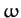. In this case, we would expect the system to eventually settle down to some steady oscillatory pattern of motion with the same frequency as the external forcing. Let us investigate the properties of this type of driven oscillation.

Suppose that our system is subject to an external force of the form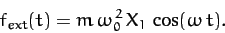(104)

Here,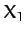is the amplitude of the oscillation at which the external force matches the restoring force, (76). Incorporating the above force into our perturbed equation of motion, (83), we obtain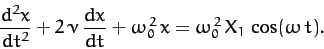(105)

Let us search for a solution of the form (84), and represent the right-hand side of the above equation as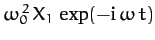. It is again understood that the physical solutions are the real parts of these expressions. Note thatis now a real parameter. We obtain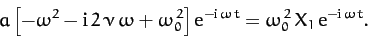(106)

Hence,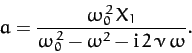(107)

In general,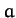is a complex quantity. Thus, we can write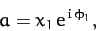(108)

where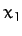and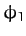are both real. It follows from Equations (84), (107), and (108) that the physical solution takes the form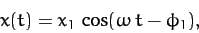(109)

where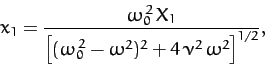(110)

and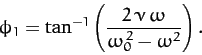(111)

We conclude that, in response to the applied sinusoidal force, (104), the system executes a sinusoidal pattern of motion at the same frequency, with fixed amplitude, and phase-lag(with respect to the external force).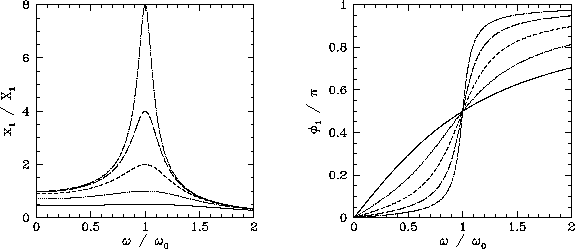Let us investigate the variation ofandwith the forcing frequency,. This is most easily done graphically. Figure 6 showsandas functions offor various values of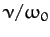. Here,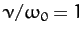,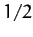,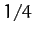,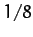, and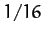correspond to the solid, dotted, short-dashed, long-dashed, and dot-dashed curves, respectively. It can be seen that as the amount of damping in the system is decreased, the amplitude of the response becomes progressively more peaked at the natural frequency of oscillation of the system,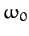. This effect is known as resonance, andis termed the resonant frequency. Thus, a weakly damped system (i.e.,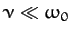) can be driven to large amplitude by the application of a relatively small external force which oscillates at a frequency close to the resonant frequency. Note that the response of the system is in phase (i.e.,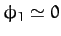) with the external driving force for driving frequencies well below the resonant frequency, is in phase quadrature (i.e.,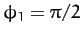) at the resonant frequency, and is in anti-phase (i.e.,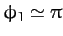) for frequencies well above the resonant frequency.

According to Equation (110),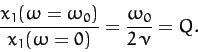(112)

In other words, the ratio of the driven amplitude at the resonant frequency to that at a typical non-resonant frequency (for the same drive amplitude) is of order the quality factor. Equation (110) also implies that, for a weakly damped oscillator (),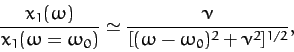(113)

provided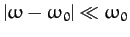. Hence, the width of the resonance peak (in frequency) is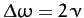, where the edges of peak are defined as the points at which the driven amplitude is reduced to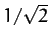of its maximum value. It follows that the fractional width is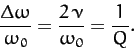(114)

We conclude that the height and width of the resonance peak of a weakly damped (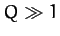) oscillator scale as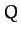and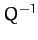, respectively. Thus, the area under the resonance curve stays approximately constant asvaries.Next: Periodic Driving Forces Up: One-Dimensional Motion Previous: Quality Factor
Richard Fitzpatrick 2011-03-31